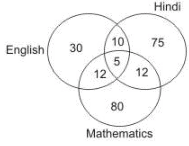Courses

# JIPMER Aptitude Mock Test 2

## 10 Questions MCQ Test JIPMER 2020: Subject wise and Full Length Mock Tests | JIPMER Aptitude Mock Test 2

Description
This mock test of JIPMER Aptitude Mock Test 2 for NEET helps you for every NEET entrance exam. This contains 10 Multiple Choice Questions for NEET JIPMER Aptitude Mock Test 2 (mcq) to study with solutions a complete question bank. The solved questions answers in this JIPMER Aptitude Mock Test 2 quiz give you a good mix of easy questions and tough questions. NEET students definitely take this JIPMER Aptitude Mock Test 2 exercise for a better result in the exam. You can find other JIPMER Aptitude Mock Test 2 extra questions, long questions & short questions for NEET on EduRev as well by searching above.
QUESTION: 1

### The given diagram shows the number of students who failed in an examination comprising papers in English, Hindi and Mathematics. The total number of students who took the test is 500. What is the percentage of students who failed in atleast two subject ?Solution:

Number of students who failed at least two subjects
= 10 + 5 + 12 + 12 = 39
Hence, required percentage = 39/ 500 x 100 = 7.8

QUESTION: 2

### A man walks northwards. After a while he turns towards his right and a little further to his left. Finally, after walking a distance of one kilometre he turns to his left again. In which direction is he moving now?

Solution: Yes option is D is correct.Actually the man walk towards "north" right and he turns right means he faces to "east" and in little minute after he turns left then he face to again in "north" direction only and after walking one kilometer he turns left means he facing "west" direction only.
QUESTION: 3

### Complete the analogous pair. 425 : 2:: 613 : ?

Solution: Like in first option middle no. is 1 just like same in second eg. middle no is 1 so and should be 1, option a)
QUESTION: 4

If the numbers from 1 to 45 which are exactly divisible by 3 are arranged in ascending order, minimum number being on the top, which would come at the ninth place from the top?

Solution:

The required numbers in ascending order are :
3, 6, 9, 12, 15, 18, 21, 24, 27, 30, 33, 36, 39, 42, 45.
If the minimum number i.e., 3 is considered to be at the top, the ninth number from the top is 27.

QUESTION: 5

Which is the Number that comes next in the series:
2, 1, (1/2), (1/4), .?

Solution:

This is a simple division series; each number is one-half of the previous number.

QUESTION: 6

Below is three statements followed by three conclusions numbered I, II, and III. You have to consider the statement and the following conclusions and decide which of the conclusions is follows in the statement :
Statement:
a. All books are students.
b. All students are houses.
c. All schools are houses.
Conclusions:
I. Some houses are schools.
II. Some students are books.
III. Some houses are books.

Solution: Option is D.correct. just write all the three words in a paper and just make a link between all the ones.
QUESTION: 7

Arrange the following in a meaningful order:
1. Pubety
3. Childhood
4. Infancy
5. Senescence

Solution:
QUESTION: 8

If 2 * 3 = 12, 3 * 4 = 20 and 4 * 5 = 30, then 2 * 6 is

Solution:

The numbers on both sides of * are increased by one and then multiplied to get the answer. 2 * 6 = 3 x 7 = 21

QUESTION: 9

In a class of 36 students the rank of Ramesh is 18th from top. What will be his rank from bottom?

Solution: If the rank of Ramesh is 18 from the top.and there are 36 students in the class. it implies that there are 18more students below him. there fore if we count from the bottom, then the student just below Ramesh from top would occupy the 18th position from bottom. hence the position of Ramesh from bottom would be 19th.
QUESTION: 10

Each question consists of four sets of three statements each. Identify the set in which the third statement follows logically from the first two.

P. All plants are moon. All moon are stars. All stars are plants.
Q. All men are thinkers. All poets are thinkers. All men write poetry.
R. Orange is a seasonal fruit. Guava is a seasonal fruit. Orange are Guavas.
S. All tubes are handles. All cups are handles. Some cups may be tubes.

Solution:

All tubes are handles ⇒ Some handles are tubes. Now All cups are handles + Some handles are tubes = No result. Because A + I = No result. However, there might be two situations which otherwise form a complementary pair, i.e., Some cups are tubes or No cup is tube. Hence, from these statements, we can conclude Some cups may be tubes. Hence (S) is valid.